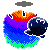## Poll

48÷2(9+3) = ??

 288 43% 43% - 40 2 56% 56% - 51 Total: 91
RDMC
Enemy Wheelbarrow Spotted..!!
+736|5863|Area 51

#### 11 Bravo wrote:

2
+286
11 Bravo
Banned
+965|4535|Cleveland, Ohio
pi
Spamtheban
Undsiputed BF2s FIFA champion of all time
+132|4141|Stoke
2
RTHKI
mmmf mmmf mmmf+1,722|6035|Oxferd Ohire
ceslayer23
+142|5659|CLOSER THAN I APPEAR
PEMDAS - parenthesis exponents multiplication/division (whichever comes first) addition/subtraction (whichever comes first)
so, 48 / 2 * (9+3)  =  48 / 2 * (12)  =  24 * 12  =  288
unnamednewbie13
Moderator
+1,975|6070|USA

The issue people have is with the implied multiplication of 2(9+3) taking precedent over 48÷. As there is no set rule requiring implied multiplication like that to take precedent over left->right multiplication or division (despite what some math books may claim without outright saying so), the biggest blunder here is not calling the answer '2,' but in the 'poor phrasing' of the problem itself. Confirmed it again (to check Dr. Peterson's confirmation) with one of my college math teachers through e-mail.

Let's break this up:

z = 48÷2(9+3)
using the distributive property, 2(9) + 2(3) = 2(12); that is to say, you get 24 either way.

However, if you plug that into the greater equation, you get this:

z = 48÷2(9+3)
We are now given a = 48; b = 2; x = 9; y = 3:
z = a÷b(x+y)
z = a÷bx+by
z = 48÷18+6
z = 2.6r + 6
z = 8.6r

What happened there? Let's try something else:

z = 48÷2(9+3)
z = a÷2(9+3)
z = a÷2(12)

Stop here for a moment, and remember that bx + by = b(x+y), but by completing a÷bx+by, you get a completely off-the-wall answer. But let's solve implied multiplication first:

z = a÷24
z = 48÷24
z = 2

There's no question that 2(9+3) separately is 24, but the two answers so far are obviously in conflict. The first thing I look at is order of operations, and here's where we encounter the double-issue of ambiguous in-line division coupled with the sketchy rule of solving for the implied multiplication b(x+y) before solving left->right multiplication or division.

So let's try interpreting it literally, without the non-standard implied multiplication precedent:

z = 48÷2(9+3)
solve for parentheses:
z = 48÷2(12)
left->right; keeping in mind that 2(12) by conventional rules does not take precedence over the 48÷:
z = 48÷2*12
48÷2 first:
z = 24*12
z = 288

Let's try solving it with just letters, once more given a = 48; b = 2; x = 9; y = 3:

0) z = a÷b(x+y)
Divide (x+y) from both sides to cancel it from the right and move it out of the way to the left:
1) z/(x+y) = a÷b
Plug in the figures and solve:
2) z/(9+3) = 48÷2
3) z/12 = 24
Multiply 12 to both sides to simplify the fraction on the left side:
4) z = 24*12
5) z = 288

Off-hand, another way to solve would be to move back to 2) and rewrite 48÷2 as a fraction and cross-multiply:

#### Code:

```    z   48
2) -- = --
12    2

3) 2z = 576
4)  z = 288```
Because it is expressed as a single-line equation, PEMDAS should be followed literally, and solved left->right by conventional standards. But the problem is this artificial language barrier promoted by sloppy textbooks. Depending on how you've been taught math, it could either look like 48/2 * 12 (literally) or 48/(2*12) (implied), and you wouldn't be at fault for believing the answer's '2.'

#### Doctor Peterson wrote:

We've dealt with the same thing here many times over the years, and I basically agree with you that it is ambiguous enough to simply avoid ever writing anything of this form.

There is no standard rule that tells you to do the multiplication first, though the rule makes sense at least visually. Some texts actually teach such a rule, but forget to tell their readers that it is not standard. The left to right approach yielding 288 is the only interpretation that fits the usual set of rules; but it is so easy to misread that I'd avoid it. The fact that mathematicians hardly ever use the in-line division symbol in the first place, using the fraction bar instead, makes it a moot point.
I would change my vote from null to 288 if I could, but null is more fitting. I really can't think of anything else to add to the thread, other than what's already been said. It's interesting, but the problem really should have been written via fractions to avoid confusion, but that's the nature of trick questions.

#### Code:

```  a
------      for 2;
b(x+y)

a
- (x+y)     for 288
b```
So if questioned further, I'm most likely just going to link back to this. "My calculator doesn't lie" depends on how it reads the rotten thing once entered.

e: Closed on request. May reopen upon a non-troll request.
Hurricane2k9
Pendulous Sweaty Balls
+1,538|5000|College Park, MD

#### unnamednewbie13 wrote:

<above-post>
can you just lock this thread? anything beyond this point is a bunch of people acting like they know more than a guy who probably has a PhD in math